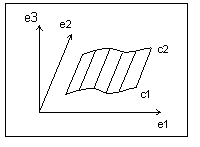Example: Ruled Surface Data Format
A ruled surface is the surface the system generates by interpolating linearly between corresponding points of two curve entities. The u coordinate is the normalized parameter at which it evaluates both curves, and the v coordinate is the linear parameter between the two points. The system does not define the curves in the local coordinate system of the part, so it must transform the resulting point by the local coordinate system of the surface. The following illustration shows a ruled surface.Data format:
 e1 (dbl) e2 (dbl) e3 (dbl) origin (dbl) Origin of the local coordinate system C1 (curve structure) First generating curve C2 (curve structure) Second generating curve
Parameterization:
(x', y', z') is the point in local coordinates
(x', y', z') = (1 - v) * C1(u) + v * C2(u)

(x, y, z) = x' * e1 + y' * e2 + z' * e3 + origin